# Rate of Change Overview

## What is Rate of Change?

Imagine you are driving in a car and that after one hour, you have traveled 30 miles. After a second hour, you have traveled an additional 45 miles, and after a third hour, 75 more miles. Say we are interested in finding out your average speed, or in other words, your rate of change, over those three hours. In this example, your average rate of change would be 50 miles per one hour. In this overview, we will discuss how to calculate the rate of change of a function, as well as how we classify those rates.

## How to Calculate Rate of Change

In order to calculate rate of change for a function $$f(x)$$, select two points $$a$$ and $$b$$ on the $$x$$-axis, where $$b$$ is greater than (or to the right of) $$a$$. We evaluate the function at those two points and take their difference, $$f(b)-f(a)$$, to determine how much height the function gains or loses. We then take the difference $$b-a$$ to determine how far we are moving along the $$x$$-axis. Finally, we divide the change in height by the change along the $$x$$-axis to calculate the ratio used for rate of change:

$$\frac{f(b)-f(a)}{b-a}=\frac{\text{change in height}}{\text{change along the x-axis}}=\text{ rate of change}$$

Notice in the following illustration that $$fa$$ and $$fb$$ represent $$y$$-values and help us determine change in height, while $$a$$ and $$b$$ are $$x$$-values which help us determine change along the horizontal $$x$$-axis.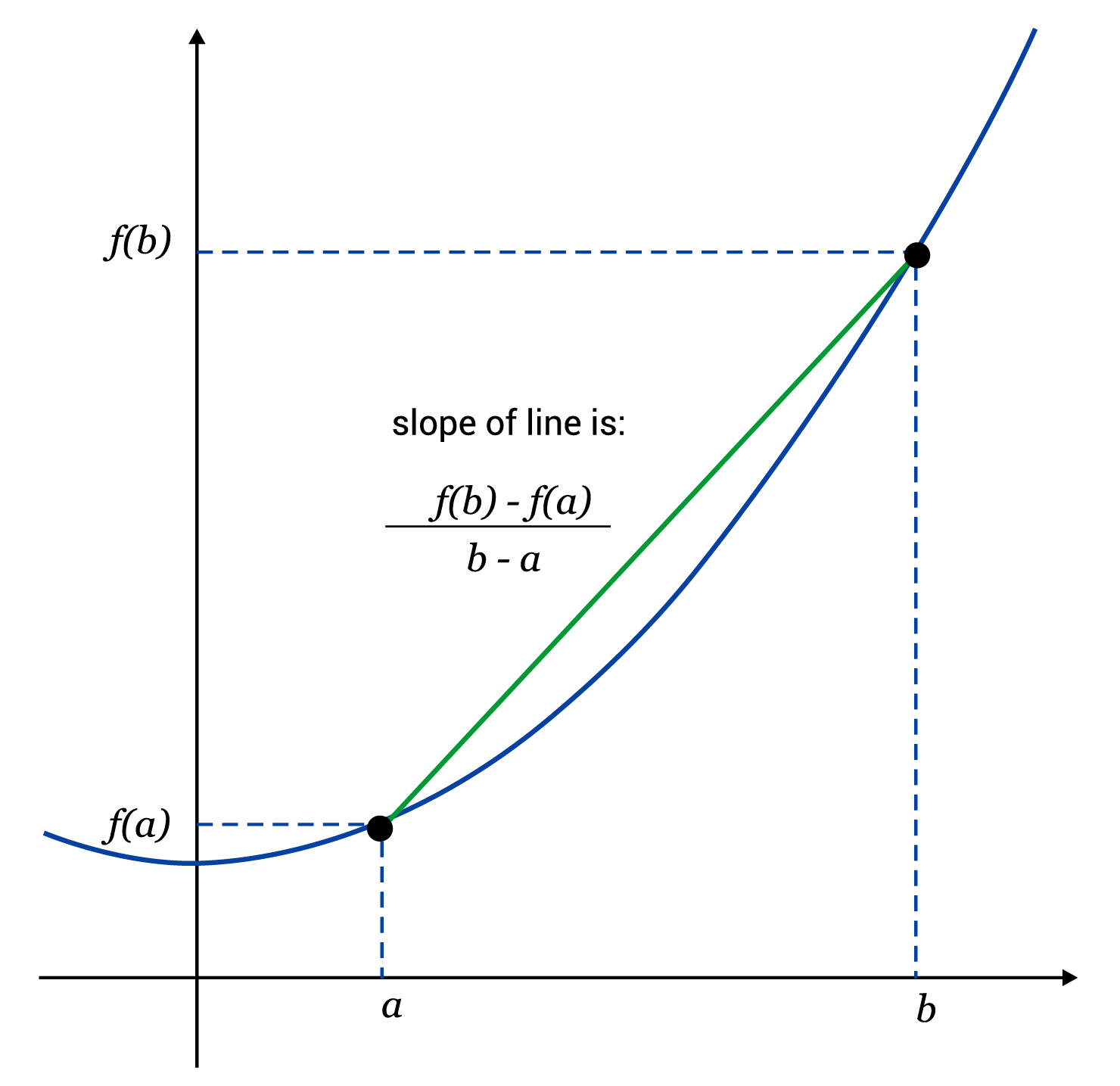### Positive, Negative, and Zero Rate of Change

Rate of change helps us to see and understand how a function is generally behaving. Is it gaining height overall? Going down? Some functions, like $$\text{sin}(x)$$ and $$\text{cos}(x)$$, which are oscillating functions, could even have zero net change! It is not surprising that we classify rates of change as positive, negative, and zero in order to indicate these general behaviors.

For example, let’s consider the function $$f(x)=x^3$$. If we select two points along the $$x$$-axis, say 0 and 2, then evaluate at those points, we get $$f(0)=0^3=0$$, and $$f(2)=2^3=8$$. In order to find the rate of change, we use the formula from above.

$$\frac{f(b)-f(a)}{b-a}=\frac{f(2)-f(0)}{2-0}=\frac{8-0}{2-0}=\frac{8}{2}=4$$

For the function $$f(x)=x^3$$, the rate of change from $$x=0$$ to $$x=2$$ is equal to 4. This means that along the section given, the function on average gains 4 units of height for each 1 unit advanced horizontally. In the image below, the blue line shows $$f(x)=x^3$$, while the yellow line depicts the rate of change. Notice that the yellow lines endpoints intersect the blue line at $$(0,0)$$ and $$(2,8)$$ and simply connect them with a straight line. If we are given a graph to begin with, we can pick out those points of intersection and use them to find the rate of change more quickly.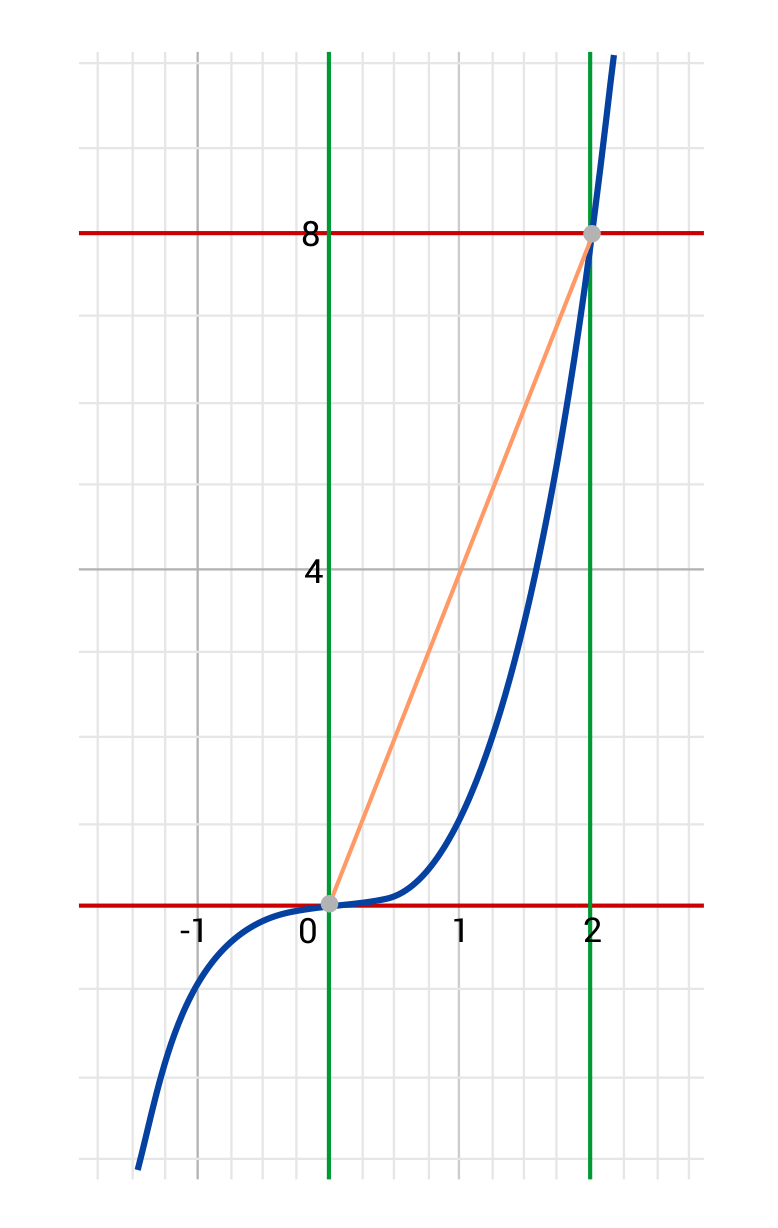From the points $$(0,0)$$ and $$(2,8)$$, $$\frac{\text{difference of y-values}}{\text{difference of x-values}}=\frac{∆x}{∆y}=\frac{8-0}{2-0}=\frac{8}{2}=4$$. It is helpful to note that when we talk about differences in x- and y-values, we frequently use the shorthand $$∆x$$ and $$∆y$$. That triangle is the uppercase Greek letter Delta, which is used to denote change. Hence, when we read “$$∆x$$”, we say either “delta $$x$$” or “change in $$x$$.”

Let’s look at another example. This time, consider the function $$f(x)=x^2$$, and compute the rates of change on the following three intervals:

• From $$x=-2$$ to $$x=0$$
• From $$x=1$$ to $$x=3$$
• From $$x=-5$$ to $$x=5$$

First, the rate of change from $$x=-2$$ to $$x=0$$ can be computed as follows:

$$\frac{f(0)-f(-2)}{0-(-2)}=\frac{0^2-(-2)^2}{2}=\frac{0-4}{2}=-2$$

This means that from $$x=-2$$ to $$x=0$$, the function $$f(x)=x^2$$ has a negative rate of change equal to -2. A negative rate of change means that a function is generally decreasing on that interval.

What about from $$x=1$$ to $$x=3$$?

$$\frac{f(3)-f(1)}{3-1}=\frac{3^2-1^2}{2}=\frac{9-1}{2}=4$$

The rate of change from $$x=1$$ to $$x=3$$ is positive, and equal to 4. This means that the function is growing on that interval.

Finally, what’s going on from $$x=-5$$ to $$x=5$$?

$$\frac{f(5)-f(-5)}{5-(-5)}=\frac{5^2-(-5)^2}{5+5}=\frac{25-25}{10}=0$$

We found an interval on which $$f(x)$$ has a zero rate of change. In other words, from $$x=-5$$ to $$x=5$$, there is a net change in height of zero. Observe the graph below and note that we found positive, negative, and zero rates of change from the same function by checking different intervals.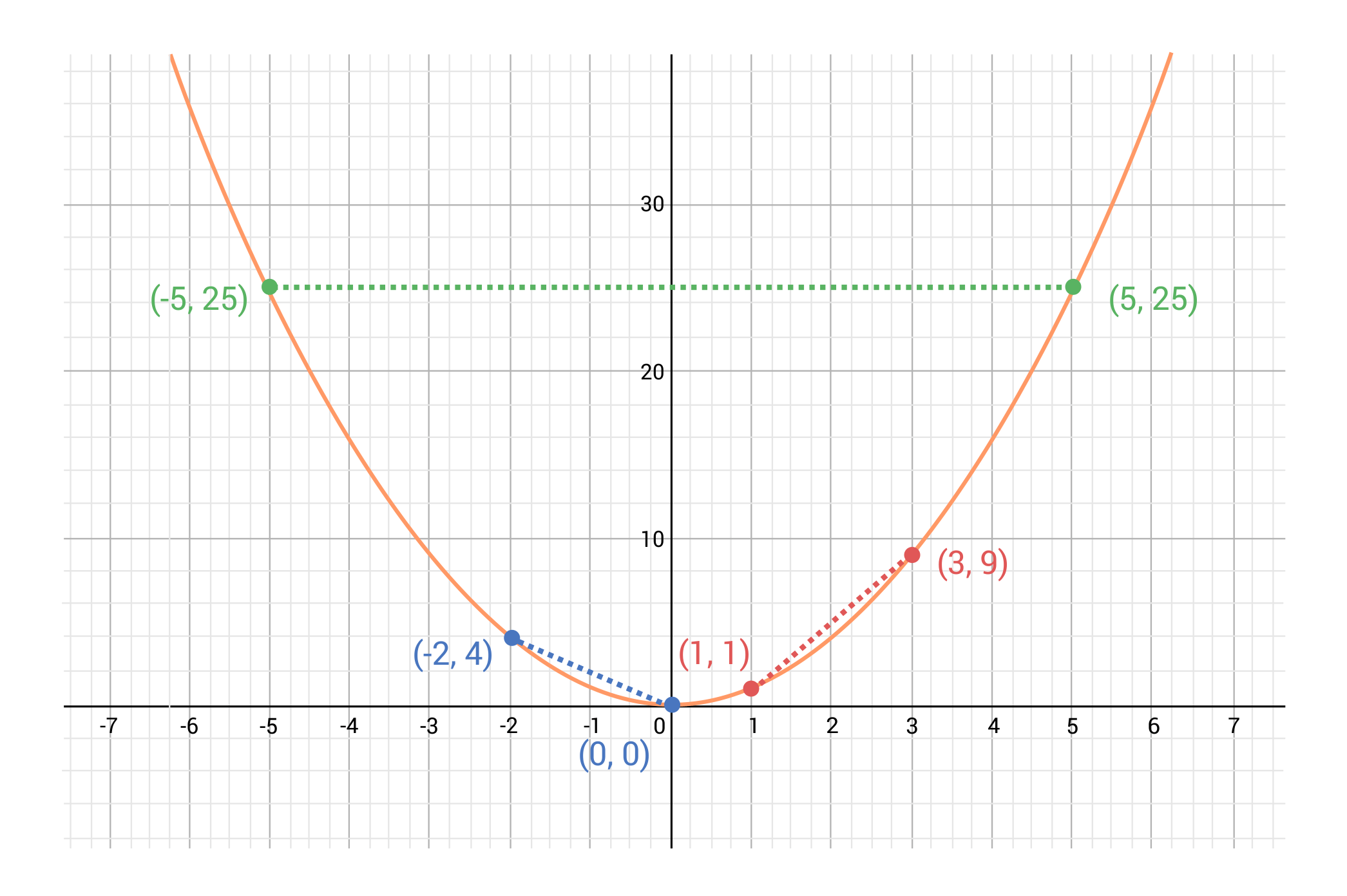## Rate of Change Sample Questions

Here are a few sample questions going over rate of change.

Question #1:

Calculate the rate of change for the function $$f(x)=3x$$ from $$x=2$$ to $$x=6$$.

The rate of change is 4

The rate of change is 3

The rate of change is 6

The rate of change is 1.5

The rate of change is 3. We can calculate this using the formula for rate of change:

$$\frac{f(6)-f(2)}{6-2}=\frac{3\times6-3\times2}{4}=\frac{18-6}{4}=\frac{12}{4}=3$$

Question #2:

Is the rate of change for the function $$f(x)=\text{cos}(x)$$ positive, negative, or zero from $$x=0$$ to $$x=2$$?

Positive

Negative

Zero

We cannot determine the answer with the information given

We can determine this by using the formula to calculate the rate of change, and then observing its sign, or by observing that the graph of $$\text{cos}(x)$$ is decreasing from $$(0,1)$$ to $$(\frac{π}{2},0)$$.

$$\frac{f(\frac{π}{2})-f(0)}{\frac{π}{2}-0}=\frac{\text{cos}(\frac{π}{2})-\text{cos}(0)}{\frac{π}{2}}=\frac{0-1}{\frac{π}{2}}=-\frac{2}{π}$$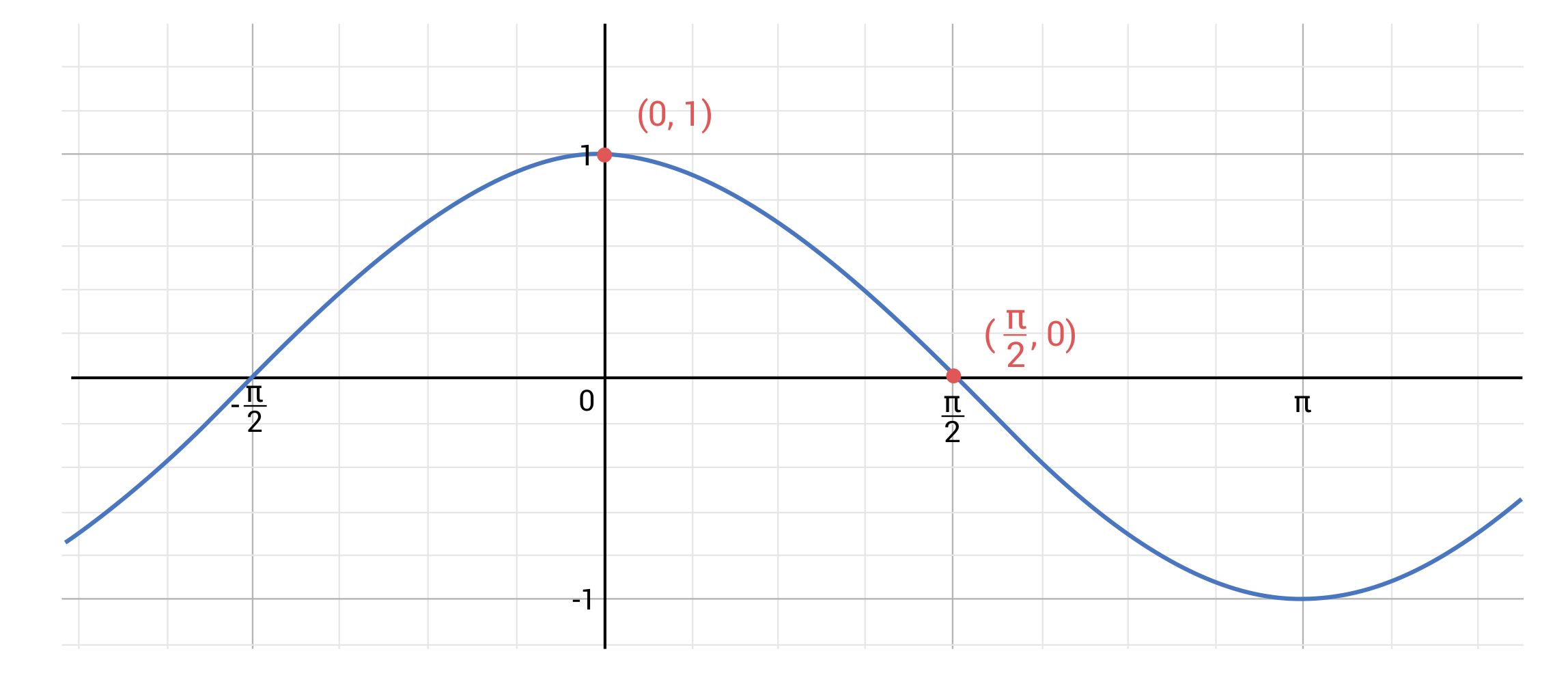Question #3:

Observe the graph given, and use $$\frac{∆y}{∆x}$$ to determine the rate of change from $$x=0$$ to $$x=2$$.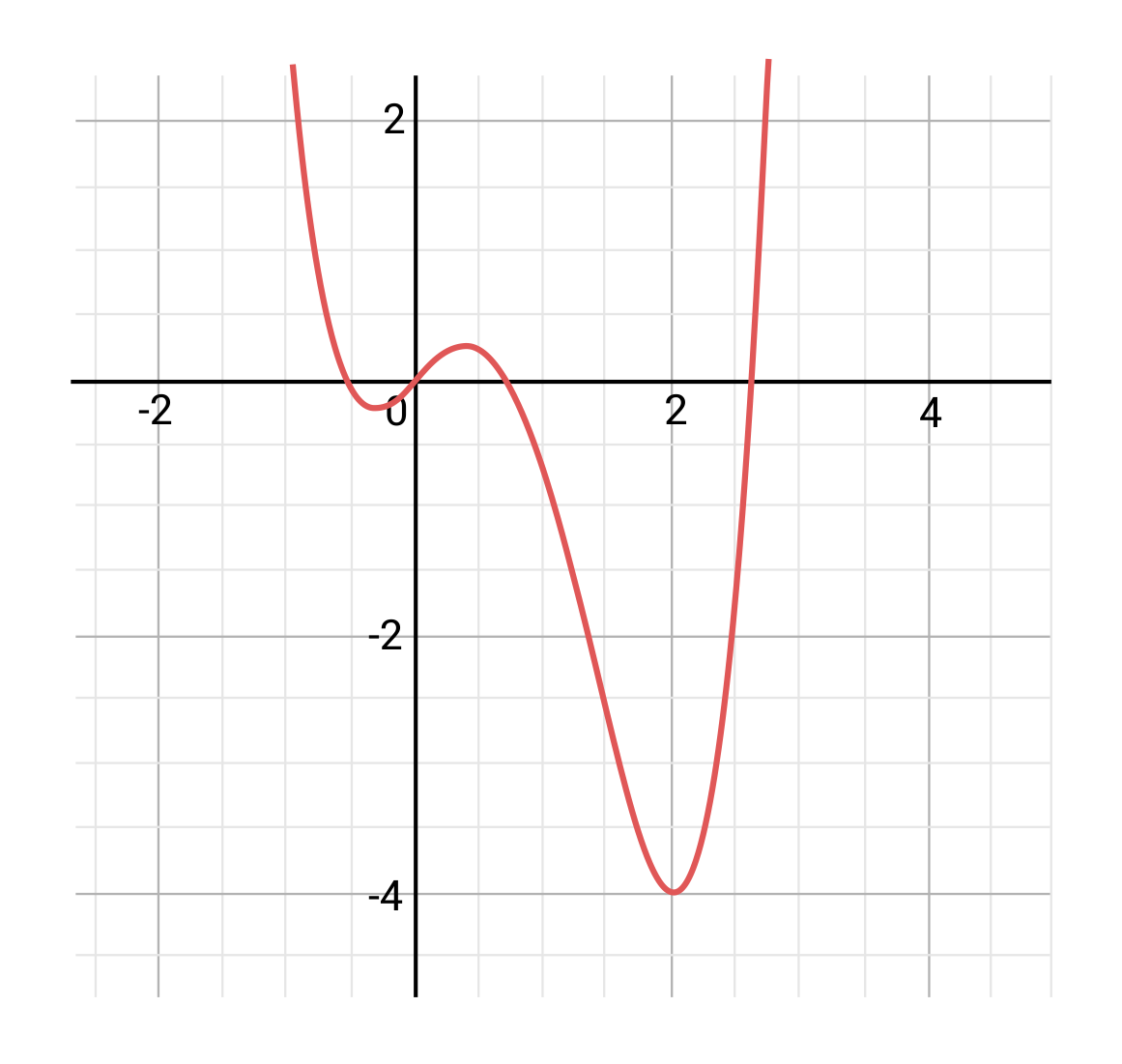Rate of change is 2

Rate of change is -3

Rate of change is -2

Rate of change is -4

We can see visually that the function travels more downward than upward on the interval from 0 to 2, which indicates that the rate of change is negative. We can also plug in the points $$(0,0)$$ and $$(2,-4)$$ that we see on our graph into the formula to determine the magnitude of the rate of change.

$$\frac{\text{difference of y-values}}{\text{difference of x-values}}=\frac{∆y}{∆x}=\frac{-4-0}{2-0}=\frac{-4}{2}=-2$$

Question #4:

What is the rate of change for the function $$f(x)=x^4+2x^2$$ from $$x=-3$$ to $$x=3$$?

The rate of change is 3

The rate of change is 9

The rate of change is -3

The rate of change is 0

We can calculate the rate of change using the formula once again:

$$\frac{f(3)-f(-3)}{3-(-3)}=\frac{(3^4+2×3^2)-((-3)^4+2×(-3)^2)}{3+3}=\frac{81+18-81-18}{6}=0$$

This result can also be seen if we graph the function: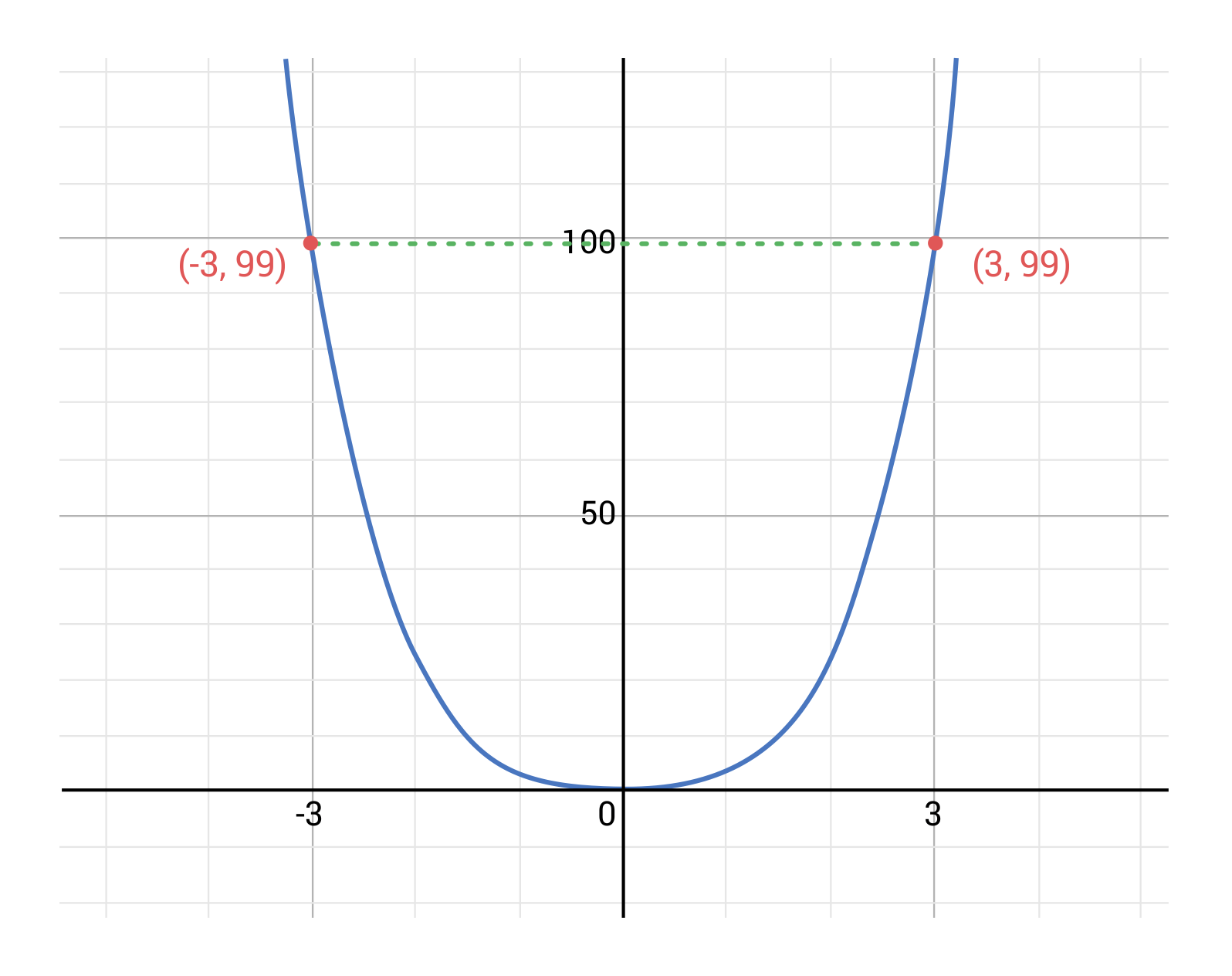Question #5:

Milly’s Restaurant is keeping track of how many customers they are serving at given times throughout the day. Today, they had 4 guests at 3 pm, then 5 guests at 4 pm, 18 guests at 5 pm, and 22 guests at 6 pm. What is the average rate of change in number of customers from 3 pm to 6 pm?

5 guests

8 guests

6 guests

10 guests

This means that on average, six more customers were present with each passing hour. We can see this by using the formula:’

$$\frac{f(6)-f(3)}{6-3}=\frac{22-4}{6-3}=\frac{18}{3}=6$$

by Mometrix Test Preparation | This Page Last Updated: September 20, 2023

Get Actionable Study Tips
Join our newsletter to get the study tips, test-taking strategies, and key insights that high-performing students use.
Get Actionable Study Tips
Join our newsletter to get the study tips, test-taking strategies, and key insights that high-performing students use!